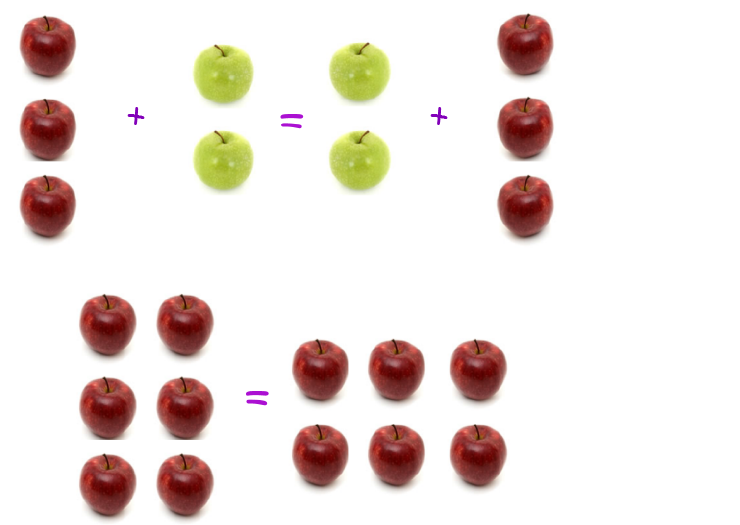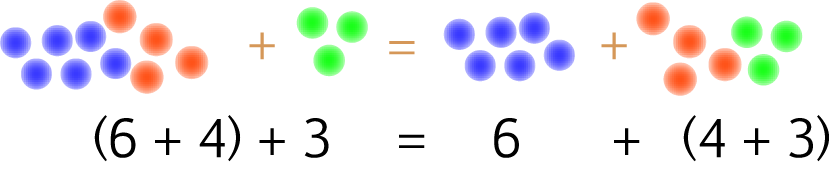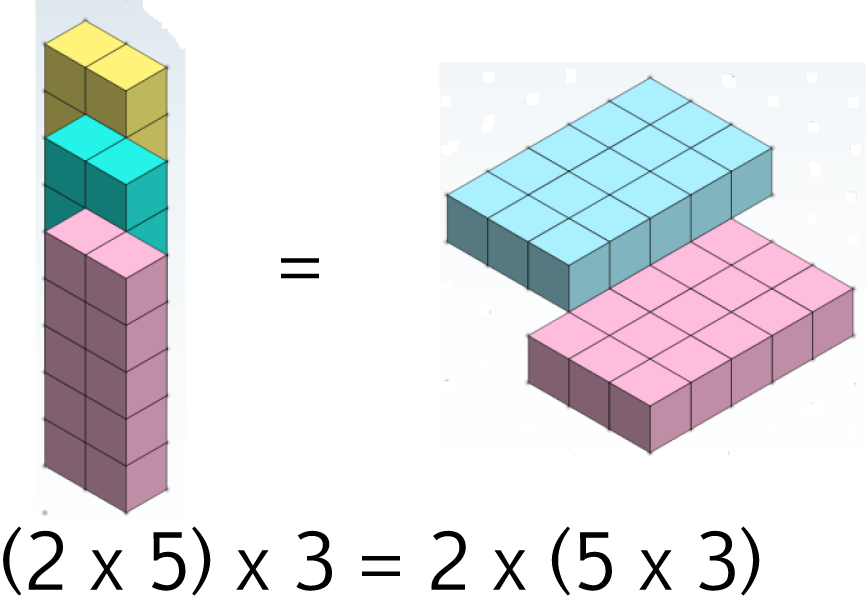# Commutative, Associative and Distributive Laws

These laws for addition and multiplication sound very complicated, but they are really very simple to use and remember.

## Commutative LawsSometimes the order you do things in is really important. For example, you would never put your socks on after your shoes. And, what were Batman and Robin thinking, putting their undies on over their tights? However, there are times when order does not matter, and that is the case with addition and multiplication. You can switch numbers around all over the place when you add and multiply. You'll always get the same answer. This is what we mean by the commutative laws for addition and multiplication.

### The Commutative Law for Addition

The commutative law for addition says that order doesn't matter when you are adding. If $a$ and $b$ are numbers, then the commutative law for addition says that

$a + b = b + a$

For example,So, $2 + 5 = 7 = 5 + 2$.

### The Commutative Law for Multiplication

There's also a commutative law for multiplication. It says that, whenever $a$ and $b$ are numbers, then

$a \times b = b \times a$

The pictures below give examples of the commutative laws for both addition and multiplicationThe top sum with apples shows that $3 + 2 = 5 = 2 + 3$. The bottom sum with applies shows that $3 \times 2$ is the same as $2 \times 3$. They are both $6$.

It's hard to remember long words like "commutative", but if you think of commuters travelling to and from work on the train, it might help you. They travel in both directions as well.

## Associative Laws

There are "associative laws" for both addition and multiplication as well. They say that the way we group numbers together doesn't matter when we're multiplying or adding them. If $a,b$ and $c$ are numbers, then the associative law for addition says that

$(a + b) + c = a + ( b + c)$

For example,shows that $(6 + 4) + 3 = 13 = 6 + (4 + 3)$. If $a,b$ and $c$ are numbers, then the associative law for multiplication says that
$(a \times b) \times c = a \times ( b \times c)$

For example,shows that $(2 \times 5) \times 3 = 30 = 2 \times (5 \times 3)$.

Together, the associative and commutative laws for addition and multiplication can really help you to simplify calculations. Sometimes it's easier to add or multiply in a different order.

For example, if you need to find $23 + 18 + 7$, it's easier to calculate $(23 + 7) + 18 = 30 + 18 = 48$. The associative and commutative laws allow you to do just that.

In multiplication, you might be asked to calculate $5 \times (23 \times 2)$. The commutative law for multiplication tells you that this is the same as $5 \times (2 \times 23)$, and the associative law for multiplication tells you that this is the same as $(5 \times 2) \times 23 = 10 \times 23 = 230$, which is much easier to compute.

## The Distributive Law

The Distributive Law tells you how to combine multiplication and addition. It lets you "distribute" multiplication over addition. If $a,b$ and $c$ are numbers, then the distributive law states that

$a \times (b + c) = a \times b + a \times c$

For example,shows that $4 \times (2 + 3) = 4 \times 5 = 20 = 8 + 12 = 4 \times 2 + 4 \times 3$.

The distributive law comes in very handy when you need to do difficult multiplications. For example, $8 \times 307 = 8 \times (300 + 7) = 8 \times 300 + 8 \times 7 = 2,400 + 56 = 2,456$.

You can also use the distributive law in reverse, to combine two more difficult sums into one easier one. For example, $6 \times 17 + 6 \times 3 = 6 \times (17 + 3) = 6 \times 20 = 120.$

We can also use the distributive law for subtraction because $a - b$ is the same as $a + (-b)$. So, for example, $5 \times 28 - 5 \times 26 = 5 \times (28 - 26) = 5 \times 2 = 10$.

Finally, you can apply the distributive law over and over again to add a long list of products. For example, $5 \times 3 + 2 \times 3 + 1 \times 3 + 2 \times 3 = (5 + 2 + 1 + 2) \times 3 = 10 \times 3 = 30.$

## Conclusion

If $a,b$ and $c$ are numbers, then

• The commutative laws state that:
$\begin{eqnarray*} a + b &= b + a\\ a \times b &= b \times a \end{eqnarray*}$
• The associative laws state that
$\begin{eqnarray*} (a + b) + c &= a + (b + c)\\ (a \times b) \times c &= a \times (b \times c) \end{eqnarray*}$
• The distributive law states that
$\begin{eqnarray*} a \times (b + c) &= a \times b + a \times c \end{eqnarray*}$

We need to be very careful when we apply these laws. The commutative and associative laws don't work for division and subtraction. For example, $6 \div 3 = 2$, but $3 \div 6 = \dfrac{1}{2}$ and $(7 - 5) - 2 = 2 - 2= 0$, but $7 - (5 - 2) = 7 - 3 = 4$.

The distributive law does work for subtraction, but it does not work for division. For example, $36 \div (3 + 9) = 36 \div 12 = 3$, but $36 \div 3 + 36 \div 9 = 12 + 4 = 16$.

It is a great idea to remember these laws. They can make your life so much easier. But remember, wearing your undies on the outside of your tights is not a good look, even for super heroes!

### Description

This mini book covers the core of Math for Foundation, Grade 1 and Grade 2 mathematics including

1. Numbers
3. Subtraction
4. Division
5. Algebra
6. Geometry
7. Data
8. Estimation
9. Probability/Chance
10. Measurement
11. Time
12. Money
13. and much more

This material is provided free of cost for Parent looking for some tricks for their Prekinder, Kinder, Prep, Year 1 and Year 2 children

### Learning Objectives

These lessons are for kids aged 4-8 with the core objective to expose their brains to concepts of addition, subtraction, division, algebra and much more.

Author: Subject Coach
You must be logged in as Student to ask a Question.